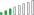cancel
Showing results for
Did you mean:Frequent Visitor

## Binning dates in cumulative total measure

Hello

I have a "Cumulative Total" measure. I want to create a table/graph where cumulative amounts are displayed against months

Simply binning the dates does not work. Then only the sum for the specific months are displayed instead of the cumulative amount.

Any tips on how i can show the cumulative total against months?

``````Cumulative Total =
CALCULATE(SUM(Fact[Amount]),
FILTER(ALL(Fact[Date]),
Fact[Date] <= MAX(Fact[Date])
)
)``````

1 ACCEPTED SOLUTIONResolver III

Hi @JakobH,

If you are looking for a cumulative total that starts again every year you can try the following:

``````Cumulative total =
CALCULATE(
SUM( Fact[Amount] ),
DATESYTD( Calendar[Date] )
)``````

where Calendar is a table containing all your dates.

Best,

2 REPLIES 2Resolver III

Hi @JakobH,

If you are looking for a cumulative total that starts again every year you can try the following:

``````Cumulative total =
CALCULATE(
SUM( Fact[Amount] ),
DATESYTD( Calendar[Date] )
)``````

where Calendar is a table containing all your dates.

Best,Frequent Visitor

Thanks for the reply, but what i wanted to achieve is to get a running total where all entries within a month are summed up.

I found a solution that works, where i create a new column which equals the the binned date group and use this column in my measure:

``````Cumulative Total =
CALCULATE(SUM(Fact[Amount]),
FILTER(ALL(Fact[BinnedDate]),
Fact[BinnedDate] <= MAX(Fact[BinnedDate])
)
)``````